# What makes music sound pleasing? Over many centuries, musicians have worked to create the "perfect" scale. It is agreed upon that the ratios for an octave and a fifth of 2: 1 and 3: 2 respectively are the most pleasing

 Introduction What makes music sound pleasing? Over many centuries, musicians have worked to create the “perfect” scale. It is agreed upon that the ratios for an octave and a fifth of 2:1 and 3:2 respectively are the most pleasing. However, these ratios cannot be used to build a complete scale without creating a discrepancy in frequencies of different octaves. This means that either some of the ratios must be adjusted slightly, which would create awkward sounding intervals, or a different system that does not use ratios must be used altogether. Composers want a broad range of capabilities so that they can be as creative as possible. They want to be able to use every degree of a scale in any key that they would want to use. Musicians want some kind of consistency so that they can play pieces by different composers without having to retune, or having a different instrument for each piece. Two approaches to the tuning problem, just and tempered tuning systems, will be explored here. The goal of each being to un-hinder musicians and composers while still allowing for the most beautiful music possible. Just Just tuning systems are based on whole number ratios (Loy 43). Just ratios are the most consonant and also are easy to work with and manipulate. The pentatonic scale is entirely based on the ratios 3:2 and 4:3 (Loy 44). This scale sounds very pleasing because it does not contain the dissonant interval of a minor second (Loy 44). The pentatonic scale gave rise to the Pythagorean scale (Loy 49). P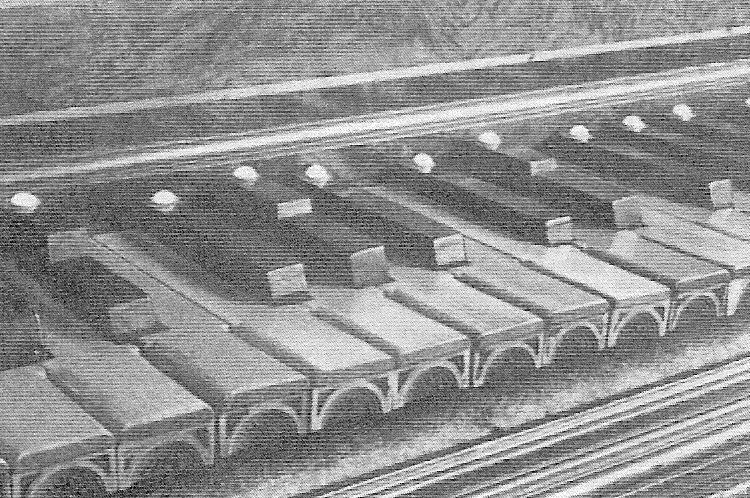ythagoras came up with the Pythagorean diatonic scale, which can be constructed using only the ratios 2:1 and 3:2. Pythagoras conducted experiments that concluded that when a string is divided and struck, it sounds most consonant when there is a low (1-4) integer ratio between the two parts of the string (Archibald). This is why he based his scale on small ratios. The scale is made from a pentatonic scale with the addition of the third and seventh scale degrees, creating two minor seconds (Loy 49). When the scale is constructed, the ratios for each individual whole tone and semitone are seen. The ratio for a whole tone being 9:8 and the ratio for a semitone being 256:243 (Loy 49). The problem with this is that going twelve fifths up from a starting note and then going seven octaves down should bring us back to the same note, however it is 1/9 of a semitone off (169). This fraction, which is the equivalent of 0.013643265, is called the Pythagorean comma (Benson 169). The intervals in the Pythagorean dodecaphonic scale, which is similar to the Pythagorean diatonic scale, are generated by raising the fifth interval to different powers (Loy 52). This gives rise to the circle of fifths (52). The Pythagorean comma appears in the scale in the different ratios for the tritones, which is the enharmonic of the augmented Picture of a piano with split keys, allowing both enharmonics to be played fourth and the diminished fifth (52). The ratio for the augmented fourth is 729:512, whereas the ratio for the diminished fifth is 1024:729 (53). Some specially made keyboards accounted for this and had split keys for the two different notes so that the correct one could be played (53). Unfortunately, both notes are necessary, because if either is left out, wolf tones, which are the dissonant sounds of a note and harmonics of a different note, occur (52).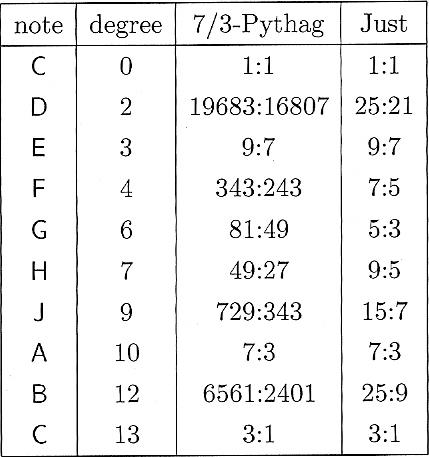In the natural chromatic scale, the major and minor thirds and sixths are off by 81/80, a ratio known as the syntonic comma (54). If the syntonic comma is distributed so that the commas from the sharp intervals are added to the flat intervals, the syntonic diatonic scale is formed (55). Unfortunately, this scale still creates wolf tones (56). The natural major scale is based on the same principles that Pythagoras utilized, but includes integers from one to six instead of one to four (60). This addition of two extra numbers creates two different ratios for the whole tone, the familiar one of 9:8 and a new ratio of 10:9 (60). The Bohlen-Pierce (B-P) scale is a unique scale that has thirteen scale degrees. Instead of octaves, the B-P scale is composed of tritaves, which is made up of a fifth added to an octave (Benson 238). Tritaves are 1901.955 cents apart (238). The ratio in the B-P scale of 7:3 is the equivalent of the fifth (239). This 7:3 is the perfect B-P tenth (239). Harry Partch created a scale with 43 scale degrees. He utilized additional prime numbers, seven and eleven to create a symmetric scale (214). The ratios are the same in either direction of the tonic, which is G for this scale (214). A ratio chart for the B-P scale J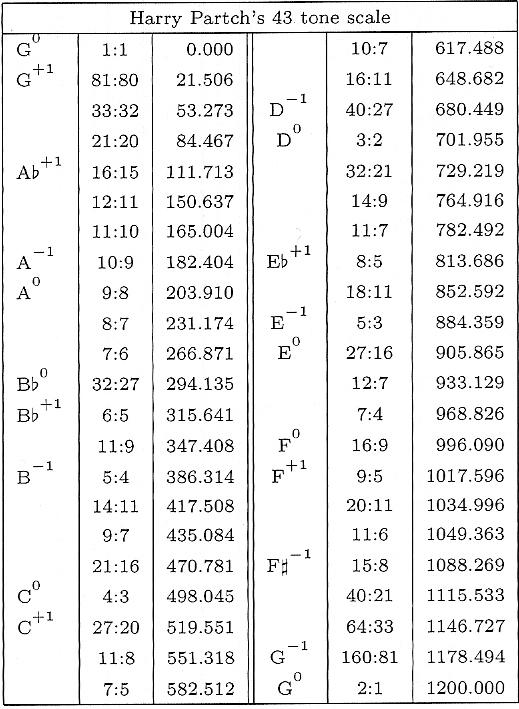ust tuning creates various problems when it comes to transposing music and in some cases even creates problems within each scale. But the ratios that just scales are founded upon create the most pleasing intervals. So to achieve this, one can either avoid transposition, add more degrees to the scale or extend the use of tempering. Tempered Tempering involves spacing notes equally throughout the octave instead of relying solely on ratios to distance notes. The octave is the only interval that does not ever change. It is the same in any scale, with the exception of the Bohlen-Pierce scale. There are three types of tempered scales: mean-tone, well-tempered and equal-tempered. Mean-tone tempering makes the perfect fifth and major third ratios close to perfect in select keys. The other ratios are not as important. The notes of the 43-tone scale The mean-tone tempered scale uses perfect major thirds for the construction of the scale (Loy 69). The goal of this scale is to have a scale that has intervals that are close to the just ratios but to also be easily transposable (69). The major thirds create irrational ratios for semitones, which makes transposition difficult. The thirds also slightly compromise the 3:2 ratio of the fifth (69). Andreas Werekmeister III created a well-tempered scale that distributed the comma among the white keys on the piano to make perfect fourths and fifths. Francesco Antonio Vallotti created a well-tempered scale that used F as a tonic. He tempered the first six fifths in the circle by 1/6 of the Pythagorean comma and left the rest as they were. Shrinking the interval of the fifth by 0.012 would make it so that 12 fifths equaled 7 octaves. T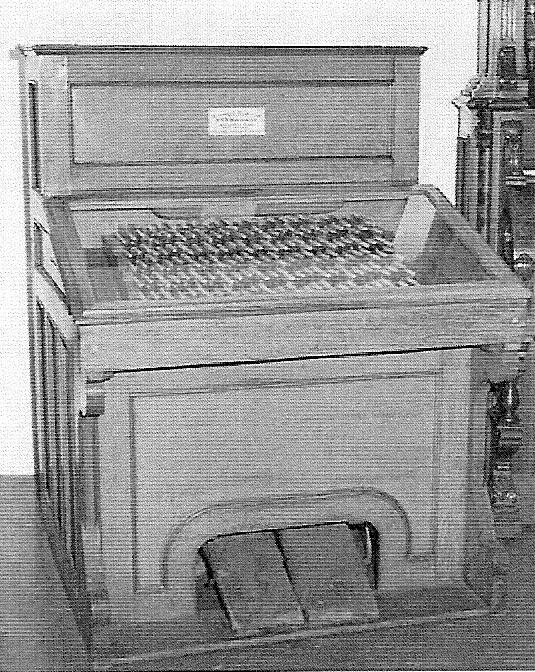here are many different kinds of equal-tempered scales. Many equal-tempered scales employ the use of microtones. Microtones are scale degrees that are less than a semitone in pitch (72). This increases the probability of finding good just ratios in the scale. The quarter tone scale is closely related to the 12 tone scale that we are accustomed to. It contains all 12 tones, plus 12 extra tones halfway between each semitone (73). Another microtone scale is the 19-tone scale. In the 19-tone scale, the ratios for thirds and sixths are improved upon and are closer to the just ratios (73). The ratios for the minor third and the major sixth are very close to pure. However, nineteen fifths and eleven octaves, which should end at the same note, are 137 cents apart, and the fifths of the scale are 0.068 cents flat, which does not make this scale superior to the chromatic equal-tempered scale. Nicolaus Mercator came up with the 53-tone scale (73). In this scale 53 fifths and 31 octaves are only 3.615 cents apart (73). A harmonium used to play the 53-tone scale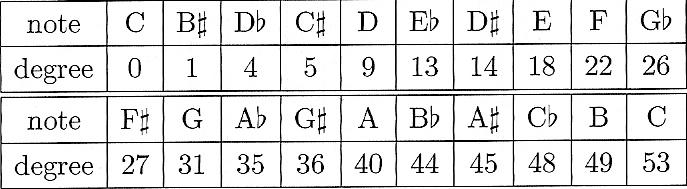A chart of notes and their 53-tone scale equivalent The tempered fifth is only 0.068 cents flat. This scale has intervals that are extremely close to just ratios. It seems that the more scale degrees there are in a scale, the more the scale will encompass just ratios. Perhaps the only “perfect” scale is the cent scale, which has 1200 degrees. Wendy Carlos created three special scales that did not have a whole number of notes in an octave (Benson 235). Her objective was to get the ratios for the major third and the perfect fifth correct (235). The three scales, named Alpha, Beta and Gamma, ranged from having just over 15 scale degrees to having just over 20 degrees (236). Conclusion The search for the perfect tuning system is a noble if not possible one. Thankfully, people like Pythagoras, Partch and Carlos have given us options for accomplishing some of the different qualities that the “perfect” scale would. As innovative as some of these options may be, they not only are possible alternatives, but also have been used in some practical sense. Thankfully, the lack of a “perfect” scale has not prevented people from composing, playing and listening to music. Works Cited Archibald, R. C. Mathematicians and Music. 2006. Mathematical Association of America. 31 May. 2007. . Benson, Dave. Music: A Mathematical Offering. Scotland, 2007 Loy, Gareth. Musimathics: The Mathematical Foundations of Music. Cambridge: MIT, 2006. .Download 0.51 Mb.Share with your friends: